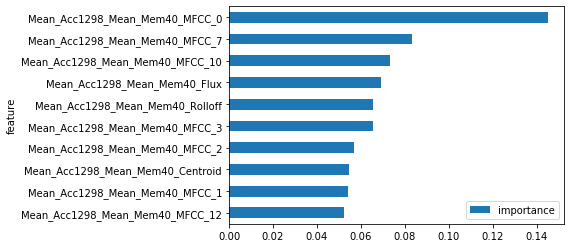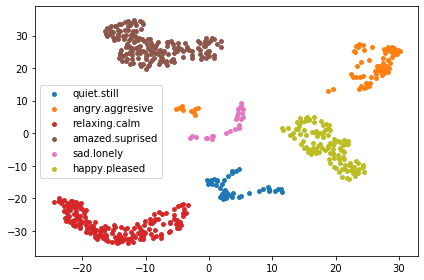# Emotions Dataset¶

This notebook walks you through a slightly more detailed example showing how a Planetoid can be used to visualise the results of an ML pipeline.

We are using the emotions dataset from OpenML which is a database of features derived from some songs that have been labelled according to their mood.

In :
import planetoids as pt
import pandas as pd
import numpy as np


## Pull the data from OpenML.¶

In order to get the proper target labels we need to follow this idmax approach.

In :
from sklearn.datasets import fetch_openml
data = fetch_openml(name='emotions', version=5, target_column=None)
df = pd.DataFrame(data.data, columns=data.feature_names)

#reverse the current one-hot nature of the targets
target = df[data.feature_names[-6:]].idxmax(1).values

#remove the target from the data
df = df.iloc[:,:-6]

#get an int representation of the target labels
b, target_int = np.unique(target, return_inverse=True)

In :
df.shape

Out:
(593, 72)

## Preprocessing¶

Use a cross-validated recursive feature elimination approach to identify the most important features using a forest of extremely randomised trees with max_depth=3

In :
from sklearn.feature_selection import RFECV
from sklearn.ensemble import ExtraTreesClassifier
estimator = ExtraTreesClassifier(n_estimators=50, max_depth=3, random_state=42)
selector = RFECV(estimator, step=1, cv=5, verbose=0)
selector = selector.fit(df.values, target)
feature_reduction = round((len(df.columns) - selector.n_features_)/len(df.columns), 2)
print('Feature Reduction: {}%'.format(100*feature_reduction))

Feature Reduction: 76.0%

In :
#print all the features with rank 1
df.columns[selector.ranking_ == 1]

Out:
Index(['Mean_Acc1298_Mean_Mem40_Centroid', 'Mean_Acc1298_Mean_Mem40_Rolloff',
'Mean_Acc1298_Mean_Mem40_MFCC_0', 'Mean_Acc1298_Mean_Mem40_MFCC_1',
'Std_Acc1298_Mean_Mem40_MFCC_4', 'Std_Acc1298_Mean_Mem40_MFCC_5',
'Std_Acc1298_Mean_Mem40_MFCC_6', 'Std_Acc1298_Mean_Mem40_MFCC_7',
'Std_Acc1298_Mean_Mem40_MFCC_9', 'Std_Acc1298_Mean_Mem40_MFCC_10',
'Std_Acc1298_Mean_Mem40_MFCC_11', 'Std_Acc1298_Std_Mem40_MFCC_3',
'Std_Acc1298_Std_Mem40_MFCC_5', 'Std_Acc1298_Std_Mem40_MFCC_6',
'Std_Acc1298_Std_Mem40_MFCC_10', 'Std_Acc1298_Std_Mem40_MFCC_11',
'BHSUM3'],
dtype='object')

Plot the 10 most important features

In :
importances = selector.estimator_.feature_importances_
pd.DataFrame(list(zip(df.columns, importances)), columns=['feature', 'importance']).set_index('feature').sort_values('importance').tail(10).plot(kind='barh')

Out:
<matplotlib.axes._subplots.AxesSubplot at 0x18cc8749668>Using the rank 1 features, reduce the dataset down to 2 dimesions

In :
from umap import UMAP #see demo notebook 2 to learn more about umap
embedding = UMAP(n_components=2,
metric='cosine',
n_neighbors=250, #focus on more global structure
angular_rp_forest=True,
target_weight=0.7,
min_dist=1.6,
random_state=42).fit_transform(df[df.columns[selector.ranking_ == 1]].values, target_int)
import matplotlib.pyplot as plt
import matplotlib.colors as colors
import matplotlib.cm as cmx

reduced = pd.DataFrame(embedding, columns=['Component1', 'Component2'])
reduced['Emotion'] = target
reduced['Emotion'] = reduced['Emotion']

x=reduced['Component2']
y=reduced['Component1']

# Get unique names of species
uniq = list(set(target))

# Set the color map to match the number of species
z = range(1,len(uniq))
cmap = plt.get_cmap('tab10')
cNorm  = colors.Normalize(vmin=0, vmax=len(uniq))
scalarMap = cmx.ScalarMappable(norm=cNorm, cmap=cmap)

# Plot each class
for i in range(len(uniq)):
indx = reduced['Emotion'] == uniq[i]
plt.scatter(x[indx], y[indx], s=15, color=scalarMap.to_rgba(i), label=uniq[i])

plt.legend()
plt.tight_layout()
plt.show()As you can see in the scatter plot above, this process has produced some nice clusters we can use to seed a new Planetoid.

We will use the Kavrayskiy VII projection, a general-purpose pseudocylindrical projection, invented by Soviet cartographer Vladimir V. Kavrayskiy in 1939.

In :
emotions = pt.Planetoid(reduced,
'Component1',
'Component2',
'Emotion')
emotions.fit_terraform(projection="kavrayskiy7",
planet_name='Emotions Demo')